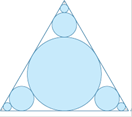### Create an Account

Home / Questions / In the figure there are infinitely many circles approaching the vertices of an equilateral...

# In the figure there are infinitely many circles approaching the vertices of an equilateral triangle,...

In the figure there are infinitely many circles approaching the vertices of an equilateral triangle, each circle touching other circles and sides of the triangle. If the triangle has sides of length 1, find the total area occupied by the circles.Apr 24 2020 View more View LessSubscribe To Get Solution